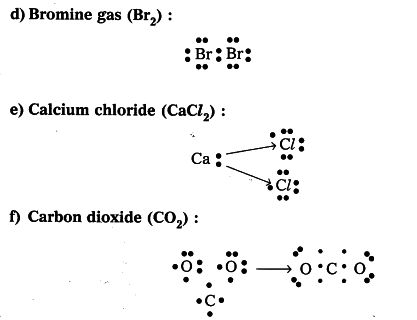# Represent each of the following atoms using Lewis notation :

Represent each of the following atoms using Lewis notation :
a) Beryllium b) Calcium c) Lithium
d) Bromine gas (\$Br _{ 2 }\$) e) Calcium chloride (\$CaCl _{ 2 }\$)
f) Carbon dioxide (\$CO _{ 2 }\$)

a) Beryllium : Be (Z = 4). Its inner shell electrons are 2 and outer shell electrons
are 2. We can represent its Lewis notation as \ddot { Be }.
b) Calcium : Ca (Z = 20). It has {{2e}^{-}} in Ist shell, {{8e}^{-}} in IInd shell and {{10e}^{-}} in IIIrd shell. So, we can represent its the electronic configuration of Ca is 2, 8, 8, 2. So its Lewis notation-is _{ . }^{ . }{ Ca }
c) Lithium : Li (Z = 3). It has {{2e}^{-}} is Ist shell and only {{1e}^{-}} in IInd shell. So its Lewis notation is Li •